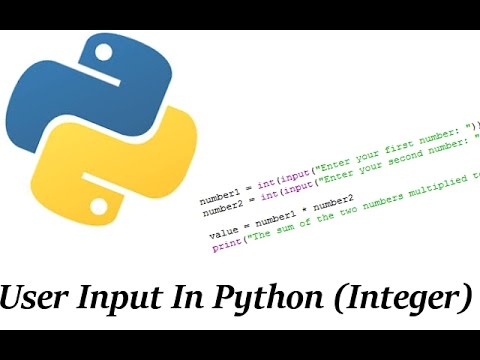# Int (input()) python

 ContentsWhat does input do?int () functionsyntaxHow to take input pyinteger input in pySummary Related articleTags

### What does input() do?

In this post we will see how to get input from user in Python. Python gives us input () function to take input. With the help of this function we can get input from user.  We have to display that in the double code of the input function. Whatever message I wrote will go in front of the user. And whatever name the user will give. That name will be stored in the variable.  You can also give another variable. The function of the input function is to accept any msg and store it in a variable.## int () Function

Now let's talk about int function in Python.  The int function has a deep connection with the input function. The int function accepts whatever value it accepts in a string.  Print to string. To solve this problem, Python has also provided us with the facility that with the help of int function we can print our string in integer. I will explain to you as much as you can understand at this time.  There will be times when we will create a program and practice as much as possible. Because programming is something that only your time wants. Today Tania will create two programs. I will use int in one program and will not use int in the other program.  And at the same time I will show you its type so that you can easily understand.

## Syntax :-

`` input([prompt])``
``````
# string input
input_a = input()

# print type
print(type(input_a))

# integer input
input_b = int(input())

# print type
print(type(input_b))

OUTPUT
30
<class 'str'>
500
<class 'int'>``````

Dear students and programmers, you don't have to worry about prompt. We call prompt a message.

Let's see the example:

``````               Program without using int() Function

a=input("enter first number : 6");

b=input("enter second number: 6");

c=a+b;

## How to take  input in Python?

In this case if the value of a&b as given by user is  6&6  respectively then the output will be 66 .As  6&6  will be treated as string and not integer.

``````Program using int() Function

a=int(input( "enter first number:6");

b=int(input( "enter second number:6");

c=a+b;

## How to take integer input in Python?

In this case if the value of  a&b as given by user is  6&6  then the output will be  12 as we  have converted the input from string to integer format.

## Summary## Example

``````
# take input from user
input_a = input()

# print data type
print(type(input_a))

# type cast into integer
input_a = int(input_a)

# print data type
print(type(input_a))

OUTPUT
300
<class 'str'>
<class 'int'>``````

## Related Articles

String in python
Remove item from a list in python
Convert User Input to a Number

## Tags

C#|Python |Python 3|pycharm |html|java|JavaScript |cpp|code|rubi|Network | Google |html5 |php|bootstrap |string |Microsoft |variable |syntax

#### 1 Comment

Today you have taught me something new. Great job. Continue to post :)Python read from standard input | by kodlogs.... Tania - Apr 17Python artificial intelligence projects for beginners Tania - May 30Python check if an elements is in a list Tania - May 28What does a percent sign mean in python Tania - May 27Number of characters in a string python Tania - May 27Different between list and array in python Tania - May 8How to count number of items in list python Tania - May 8How to increment value in dictionary python Tania - May 8str -python-example Tania - May 7How to check if a variable is an integer in python | by kodlogs Tania - May 4